# Rault’s Law

Rault’s Law: Rault’s law states that at any particular constant temperature, partial pressure of one component of mixture is equal to mole fraction of that component multiplied by its vapor pressure in its pure form (state) at this temperature (saturation temperature).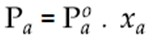Where,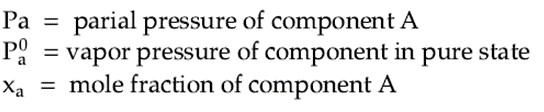## Rault’s law for binary solution: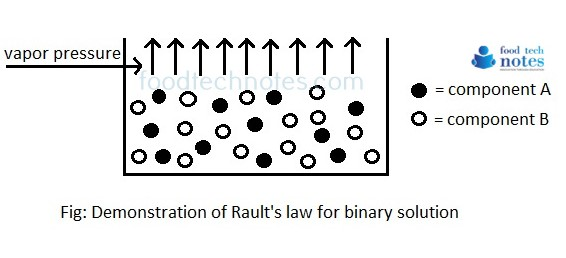Let us consider a solution containing two miscible component ‘A’ and ‘B’. The partial pressure of A and B is given by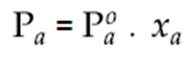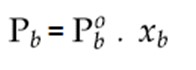By Dalton’s law of partial pressure, total pressure is obtained by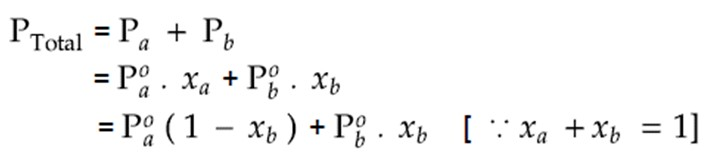This equation can be graphically represented as below.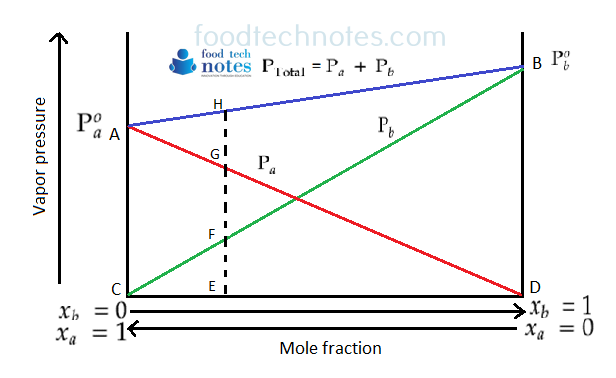According to Rault’s law, partial pressure of component varies linearly from zero to final vapor pressure as its mole fraction varies from zero to unity. If we have a pure component A, and we keep adding component B, the mole fraction of B in solution will increase from 0 to 1 and while doing so, vapor pressure of B will increase from C to B and vapor pressure of A will decrease from P°(pure state) to D. the total pressure of solution will be given by line AB. For instance, the compound anywhere at E mole fraction, vapor pressure of component A is given by ‘EG’, component B by ‘EF’ and compound total pressure by EH.

It is known that ideal mixtures follow Rault’s law according to which partial pressure is proportional to mole fraction of constituents in vapor phase. If YA and YB be mole fraction in vapor,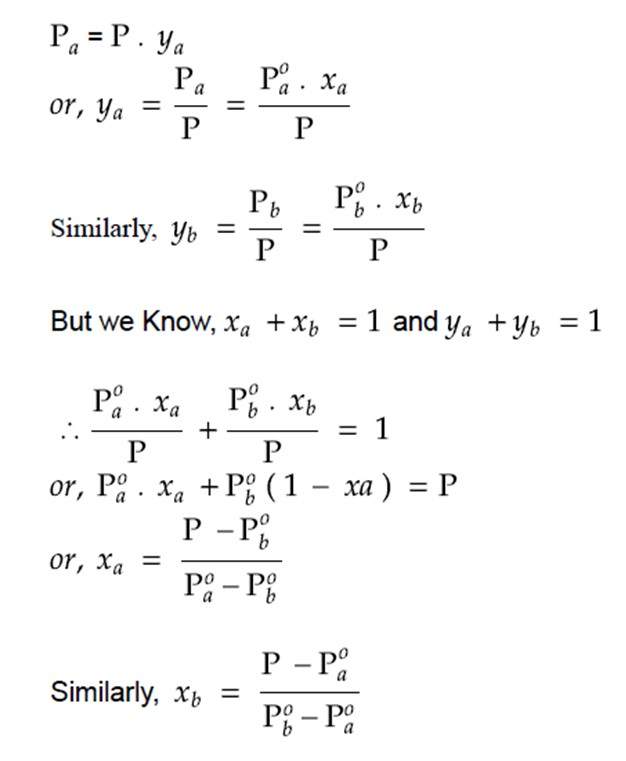For non-volatile solute, if one of the component, let us say P°B is non-volatile solute in the mixture of solution, we can say P°B = 0. In this condition, total pressure becomes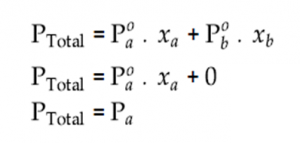This implies, in this condition, total pressure equals to partial pressure of component A.

1. Applicable to only concentrated solutions
2. Applicable for ideal mixture (i.e. which gives normal boiling point curve)
3. No chemical reaction should take place between two components